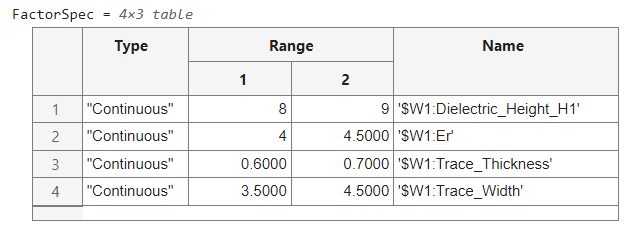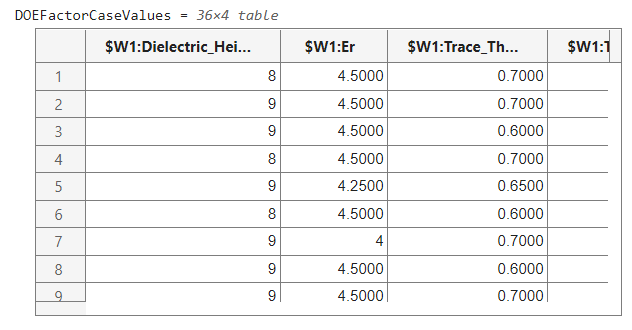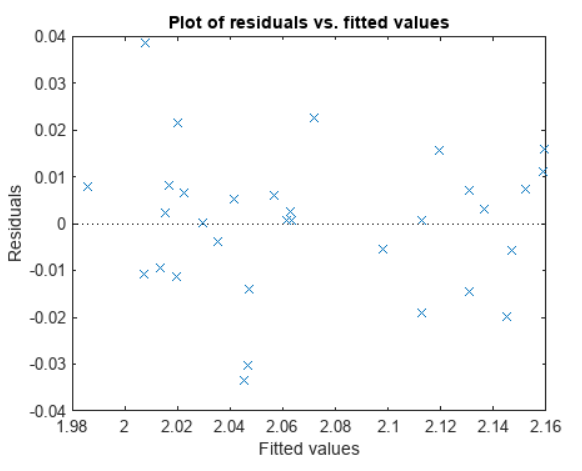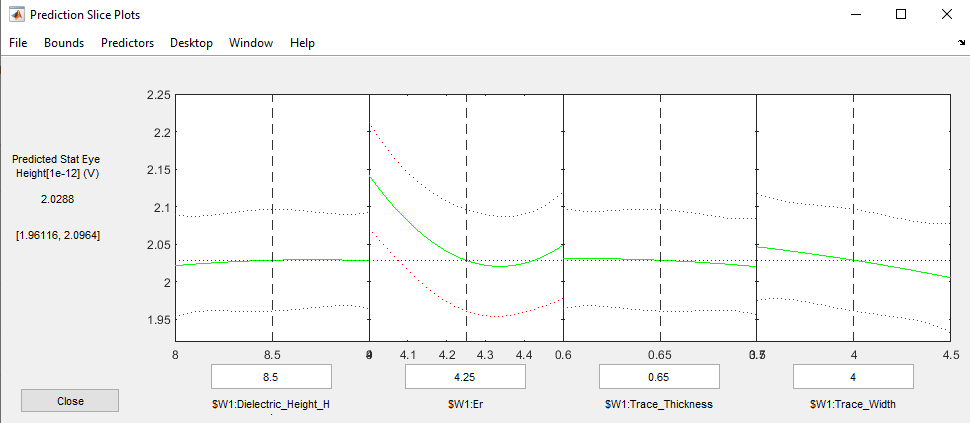# Explore Signal Integrity Interface with Design of Experiments and Response Surface Modeling

This example shows how to use the MATLAB scripting interface to Signal Integrity Toolbox™ to setup and create a Design Of Experiments (DOE). You can simulate dozens of cases and use the Statistical and Machine Learning Toolbox™ to fit and analyze a linear model.

Exploring a solution space with a large number of variables each with multiple possible values requires a lot of compute resources and time. It is not typically feasible to exhaustivly simulate every factor combination. Alternatively, when you are searching for the best or worst case factor conditions, sweeping one variable at a time can lead to sub-optimal optimization due to local maximums and minimums. A well established methodology, DOE and Response Surface Modeling (RSM), is a powerful way to sample the solution space and fit a polynomial model to the data. You can use the resulting model to predict the best or worst case conditions or leveraged as a surrogate model for advanced analysis.

This example is for the user that has familiarity with both Serial Link Designer and Design of Experiments. Other examples and resources are available for those new to Design of Experiments and Serial Link Designer.

Design of Experiments (DOE) - MATLAB & Simulink (mathworks.com)

Analyze Backplane with Line Cards - MATLAB & Simulink (mathworks.com)

### Open Signal Integrity Toolbox Project

For this experiment, use a 3 inch differential stripline transmission line (Tline) and configure the stripline properties of dielectric height, trace width, trace thickness, and dielectric constant to results in the best system performance. The attached compressed folder contains the Serial Link Designer project '`DOETLine'` which is already configured to allow the varying of these solution space variables.

Serial Link Designer projects have several levels of folders and on Windows operating systems the resulting path length can exceed the maximal character requirements and can result in confusing error messages. Therefore, for Windows operating systems, create the directory 'C:\SLD' and copy the example files there. Unzip the file 'DOETLine.zip' and open the file 'DOETlineExampleRunMe.mlx'. This file 'DOETlineExample.mlx' will not run any of the MATLAB code below and is for documentation purposes only.

```%Open Signal Integrity Project sip = SignalIntegrityProject('DOETLine',opendesigner=true); %Put in non-case mode (permutation mode) sip.Interfaces(1).Sheets(1).CaseMode = false; ```

### Get Solution Space Variables that Change

For this example, DOE factors are defined by setting solution space variables to more than one value in the non-case mode (permutation mode) solution space. Observe how three value columns are defined for variables Dielectric_Height_H1, Er, Trace_Thickness and Trace_Width. The code below searches the solution space and identifies the varying factors, their ranges and variable type.

When applying this methodology to your own project, setup Serial Link Designer with the settings and options you want to use. Give variables more than one values to identify them as DOE factors. If your project uses Variation Groups, you ned to modify the script to ensure that these connected variables vary together.

```%Get Solution Space SolutionSpaceTable = sip.Sheets(1).SolutionSpace.Table; %Variable names variableNames = SolutionSpaceTable.Properties.VariableNames; %Find Value columns that start with Value_ valueColumnsIndex = ~cellfun(@isempty,regexp(variableNames,'^Value_')); %Figure out which Value colunmns have multiple values valueColumns = SolutionSpaceTable(:,valueColumnsIndex); rowsWithMoreThanOneValue = sum(~ismissing(valueColumns),2)>1; %Define DOE variable as Solution Space variables with more than one value %column. doeVariables = SolutionSpaceTable(rowsWithMoreThanOneValue,:); doeVariablesArray = doeVariables.Variables; doeVariablesArrayValues = doeVariablesArray(:,valueColumnsIndex); NumberOfVariables = height(doeVariables); %Define Factor specification for DOE FactorSpec = cell(NumberOfVariables,3); unitStr = cell(NumberOfVariables,1); for ii = 1:NumberOfVariables %Variable Name FactorSpec{ii,3} = doeVariables.Variable{ii}; if strcmpi('Soft Range',doeVariables.Format(ii)) FactorSpec{ii,1} = "Continuous"; %Variable Type %Get set of possible values variableSet = unique(doeVariablesArrayValues(ii,:)); variableSet(ismissing(variableSet)) = []; %Determine unit unitStr{ii} = regexp(variableSet(1),'(mil|in)','match','once'); %Strip out unit string vs2 = regexprep(variableSet,'(mil|in)\$',''); vs3 = str2double(vs2); %Define Variable range if isnan(vs3(1)) tmp = sprintf('"%s" ',vs2); error('%s unable to be converted to double.',tmp) %If this happends, perhaps the above unit search and replace %needs to be expanded to include new units. else FactorSpec{ii,2} = [min(vs3),max(vs3)]; %Variable Range end elseif strcmpi('List',doeVariables.Format(ii)) %Get set of possible values variableSet = unique(doeVariablesArrayValues(ii,:)); variableSet(ismissing(variableSet)) = []; if isnan(str2double(variableSet{1})) FactorSpec{ii,1} = "Categorical"; %Variable Type FactorSpec{ii,2} = cellstr(variableSet); %Variable Range else FactorSpec{ii,1} = "Discrete Numeric"; %Variable Type variableSet = str2double(variableSet); FactorSpec{ii,2} = variableSet; %Variable Range end else error('Solution Space Format %s Unknown.',doeVariables.Format(ii)) end end %Create Factor Specification table FactorSpec = cell2table(FactorSpec, "VariableNames", ... ["Type", "Range", "Name"]) ```Continuous factors represent variables that can take any numeric value between its range. Categorical factors are for string like variables like Corner which can have values of 'SS', 'TT' or 'FF'. Discrete Numeric factors are for numerical variables that can only take certain values likes integers, 0, 1, 2, 3, etc.

### Create Design

After identifying the factors, use design of experiments to sample the solution space to increase the chances of obtaining a good model fit for a quadratic model including terms for the constant, linear, interaction, and squared terms.

```%Determine the number of runs if iscell(FactorSpec.Range) nruns = sum(cellfun(@numel, FactorSpec.Range)) + 30; else n = height(FactorSpec); nruns = 2^n + 20; end ntries = 5; model = 'quadratic'; %Quadratic: Constant, linear, interaction and squared terms DOEFactorCaseValues = makedesign(FactorSpec,nruns,'NumTries',ntries,'Model',model) ```### Write DOE Factor Cases to Solution Space

After identifying the DOE factor values, update the Serial Link Designer solution space.

```%Enable Case Mode sip.Interfaces(1).Sheets(1).CaseMode = true; %Reset Case Mode so there is only one case. Use the first column in %non-case mode (permutation mode) as the default. varNames = SolutionSpaceTable.Variable; for ii = 1:length(varNames) sip.Interfaces(1).Sheets(1).SolutionSpace.updateSolutionSpace(... varNames(ii),SolutionSpaceTable.Value_1(ii)); end %Set Design Of Experiments variable values for ii = 1:width(DOEFactorCaseValues) %Define unit if isempty(unitStr{ii}) || any(ismissing(unitStr{ii})) varUnit = ''; else varUnit = unitStr{ii}; end %Determine case value string if isempty(varUnit) caseValues = string(DOEFactorCaseValues{:,ii}); else caseValues = strings(1,height(DOEFactorCaseValues)); for jj = 1:nruns caseValues(jj) = sprintf('%s%s',string(DOEFactorCaseValues{jj,ii}),varUnit); end end %Update Solution Space for each DOE Factor sip.Interfaces(1).Sheets(1).SolutionSpace.updateSolutionSpace(... string(FactorSpec.Name{ii}),caseValues); end ```

### Run Simulations

Run the simulations.

```%Ensure parallel simulations are used sip.Parallel = true; %Ensure Time Domain Analysis is off and Statistical Analysis is on sip.simulationSetup("includeStatisticalAnalysis",true,"includeTimeDomainAnalysis",false); %Run! sip.run ```

### Statistical Simulation Results

When the simulations complete, gather the results. Use the statistical eye height at a BER of 1e-12 as the system performance meteric. Combine this with the DOE factor values and fit a linear quadratic model to the data. When fitting any model to data, it is essential to be wary of and avoid over-fitting. Always look for the simplest model that explains the data adequately.

```%Get statistical simulation results ResultsTable = sip.Interfaces(1).Sheets(1).Results; %Get Eye height data hdr = ResultsTable.Properties.VariableNames'; ehColumn = find(strcmp(hdr,'Stat Eye Height[1e-12] (V)')); eh = ResultsTable(:,ehColumn); %Combine DOE Factors values with system response (eye height) tableForFitting = [DOEFactorCaseValues,eh]; %Fit linear model. Ideally use the same model for fitting as used in the %DOE case determination above. lm = fitlm(tableForFitting,'quadratic'); ```

### Visualize Fit

The Actual vs. Predicted plot is a great summary of the fit performance. A perfect fit will result in all observation cases laying on the perfect line. R-squared is a metric that measures the variation within a regression model and is ideally 1 and at worst 0. R-squared adjusted is a modified R-squared metric which penalizes for including irrelevant predictors in the model.

```%Create Actual vs Predicted plot ypred = predict(lm,DOEFactorCaseValues); %Predicted response actual = eh{1:end,1}; %Actual response %Determine range of perfect predition line minv = min([ypred;actual]); maxv = max([ypred;actual]); perfect = [minv,maxv]+(maxv-minv)*0.05*[-1 1]; %Visualize plot(actual,ypred,'o',perfect,perfect), grid on axis equal legend('Observations','Perfect prediction','location','best') xlabel('Actual response') ylabel('Predicted response') title(sprintf('Linear model fit for %s\nRMSE: %g, R^2: %g, R^2 Adjusted: %g',... lm.ResponseName,lm.RMSE,lm.Rsquared.Ordinary,lm.Rsquared.Adjusted)) ```An R-squared value of 0.95 and R-squared adjusted of 0.90 or greater is typically an indication of a good fit for signal integrity simulation model fits. This fit could be improved by eliminating uncontrolled parameters (such as the adaptive CTLE and the adaptive DFE) or by decreasing the range of each factor.

### Visualize Residuals

A residual is the difference between the actual and predicted performance. A residual plot that contains some structure and is not randomly distributed is an indication that more predictors could be used to improve the model fit. The residual plot below appears random and does not contain exploitable struture.

```plotResiduals(lm,'fitted'); ```### View Prediction Slice Plots

Use the prediction slice plot to visualize the profile of a multi-dimensional continuous model. Click and drag the vertical dashed line for each factor to explore the factor space and find the combination of factors that maximize system performance. For this fit, minimizing the dielectric constant 'Er', minimizing the trace width, maximizing the dielectric height H1 and setting the trace thickness to 0.64 results in the predicted maximum system performance.

```plotSlice(lm) ```### Close Signal Integrity Project

```sip.exit ```

### Next Steps

Explore further by modifying the factor ranges, by adding other factors to the fit and by using other fit models. The Statistics and Machine Learning Toolbox's Regression Learner App is very powerful in its ability to explore the effectiveness of a large variety of models.

Additionally, take the predicted best/worst case factor values and simulate them to double check that the actual predicted value is contained within the error bars of the fit.

### References

How Design of Experiments Saved my CEI VSR 28G Design | 2016-09-18 | Signal Integrity Journal

#### Helper functions:

```function t1 = makedesign(FactorSpec,NumRuns,NVArgs) %Function to make a design from a specification table arguments FactorSpec NumRuns NVArgs.NumTries {mustBePositive,mustBeInteger} = 1 NVArgs.Model = 'linear' end nfactors = height(FactorSpec); [bounds,iscat] = makebounds(FactorSpec,NVArgs.Model); d1 = rowexch(nfactors, NumRuns, NVArgs.Model, "Bounds",bounds, "Tries",NVArgs.NumTries, "Categorical",iscat); t1 = array2table(d1,"VariableNames",FactorSpec.Name); t1 = applylevels(t1,FactorSpec); end function [bnds,iscat] = makebounds(spec,model) % Function to make the 'bounds' input from the specification table nfactors = height(spec); range = spec.Range; type = spec.Type; if isnumeric(range) bnds = cell(size(range,1),1); for ii = 1:size(range,1) bnds{ii} = range(ii,:); end range = bnds; else bnds = range; end iscat = false(1,nfactors); for j = 1:nfactors switch(type{j}) case "Continuous" % do error checking if model=="linear" bnds{j} = 0:1; else bnds{j} = [0 .5 1]; end case "Discrete Numeric" % use group numbers, fix up later bnds{j} = 1:numel(range{j}); case "Categorical" % use group numbers, fix up later bnds{j} = 1:numel(range{j}); iscat(j) = true; end end iscat = find(iscat); end function t = applylevels(t,spec) %Function to convert standard values to the specified ranges or sets for each factor nfactors = height(spec); if isnumeric(spec.Range) range = cell(size(spec.Range,1),1); for ii = 1:size(spec.Range,1) range{ii} = spec.Range(ii,:); end else range = spec.Range; end type = spec.Type; for j = 1:nfactors vname = t.Properties.VariableNames{j}; vals = range{j}'; switch(type{j}) case "Continuous" x = t.(vname); x = vals(1) + x*diff(vals); case "Discrete Numeric" x = vals(t.(vname)); case "Categorical" x = vals(t.(vname)); end t.(vname) = x; end end ```